# Resources tagged with: Place value

Filter by: Content type:
Age range:
Challenge level:

### There are 83 results

Broad Topics > Numbers and the Number System > Place value### Napier's Bones

##### Age 7 to 11 Challenge Level:

The Scot, John Napier, invented these strips about 400 years ago to help calculate multiplication and division. Can you work out how to use Napier's bones to find the answer to these multiplications?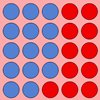### Place Value as a Building Block for Developing Fluency in the Calculation Process

##### Age 5 to 11

This article for primary teachers encourages exploration of two fundamental ideas, exchange and 'unitising', which will help children become more fluent when calculating.### Six Is the Sum

##### Age 7 to 11 Challenge Level:

What do the digits in the number fifteen add up to? How many other numbers have digits with the same total but no zeros?### Spell by Numbers

##### Age 7 to 11 Challenge Level:

Can you substitute numbers for the letters in these sums?### ABC

##### Age 7 to 11 Challenge Level:

In the multiplication calculation, some of the digits have been replaced by letters and others by asterisks. Can you reconstruct the original multiplication?### Being Resourceful - Primary Number

##### Age 5 to 11 Challenge Level:

Number problems at primary level that require careful consideration.##### Age 5 to 11 Challenge Level:

Try out this number trick. What happens with different starting numbers? What do you notice?### Being Collaborative - Primary Number

##### Age 5 to 11 Challenge Level:

Number problems at primary level to work on with others.### Alien Counting

##### Age 7 to 11 Challenge Level:

Investigate the different ways these aliens count in this challenge. You could start by thinking about how each of them would write our number 7.### Trebling

##### Age 7 to 11 Challenge Level:

Can you replace the letters with numbers? Is there only one solution in each case?### All the Digits

##### Age 7 to 11 Challenge Level:

This multiplication uses each of the digits 0 - 9 once and once only. Using the information given, can you replace the stars in the calculation with figures?### One Million to Seven

##### Age 7 to 11 Challenge Level:

Start by putting one million (1 000 000) into the display of your calculator. Can you reduce this to 7 using just the 7 key and add, subtract, multiply, divide and equals as many times as you like?### Being Resilient - Primary Number

##### Age 5 to 11 Challenge Level:

Number problems at primary level that may require resilience.### Multiply Multiples 2

##### Age 7 to 11 Challenge Level:

Can you work out some different ways to balance this equation?### Multiply Multiples 1

##### Age 7 to 11 Challenge Level:

Can you complete this calculation by filling in the missing numbers? In how many different ways can you do it?### Oddly

##### Age 7 to 11 Challenge Level:

Find the sum of all three-digit numbers each of whose digits is odd.### Multiply Multiples 3

##### Age 7 to 11 Challenge Level:

Have a go at balancing this equation. Can you find different ways of doing it?### Number Detective

##### Age 5 to 11 Challenge Level:

Follow the clues to find the mystery number.##### Age 11 to 14 Challenge Level:

Replace the letters with numbers to make the addition work out correctly. R E A D + T H I S = P A G E### Coded Hundred Square

##### Age 7 to 11 Challenge Level:

This 100 square jigsaw is written in code. It starts with 1 and ends with 100. Can you build it up?### Round the Three Dice

##### Age 7 to 11 Challenge Level:

What happens when you round these three-digit numbers to the nearest 100?##### Age 5 to 11 Challenge Level:

Who said that adding couldn't be fun?### Diagonal Sums

##### Age 7 to 11 Challenge Level:

In this 100 square, look at the green square which contains the numbers 2, 3, 12 and 13. What is the sum of the numbers that are diagonally opposite each other? What do you notice?### Journeys on the Gattegno Tens Chart

##### Age 5 to 11

Alf describes how the Gattegno chart helped a class of 7-9 year olds gain an awareness of place value and of the inverse relationship between multiplication and division.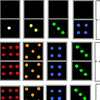### Ordering

##### Age 5 to 11

This set of activities focuses on ordering, an important aspect of place value.### Which Is Quicker?

##### Age 7 to 11 Challenge Level:

Which is quicker, counting up to 30 in ones or counting up to 300 in tens? Why?### Nice or Nasty for Two

##### Age 7 to 14 Challenge Level:

Some Games That May Be Nice or Nasty for an adult and child. Use your knowledge of place value to beat your opponent.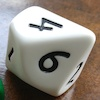### Less Is More

##### Age 5 to 11 Challenge Level:

Use your knowledge of place value to try to win this game. How will you maximise your score?### Football Sum

##### Age 11 to 14 Challenge Level:

Find the values of the nine letters in the sum: FOOT + BALL = GAME### Cayley

##### Age 11 to 14 Challenge Level:

The letters in the following addition sum represent the digits 1 ... 9. If A=3 and D=2, what number is represented by "CAYLEY"?### Two and Two

##### Age 11 to 16 Challenge Level:

How many solutions can you find to this sum? Each of the different letters stands for a different number.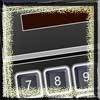### Calculator Bingo

##### Age 7 to 11 Challenge Level:

A game to be played against the computer, or in groups. Pick a 7-digit number. A random digit is generated. What must you subract to remove the digit from your number? the first to zero wins.### What Do You Need?

##### Age 7 to 11 Challenge Level:

Four of these clues are needed to find the chosen number on this grid and four are true but do nothing to help in finding the number. Can you sort out the clues and find the number?### Becky's Number Plumber

##### Age 7 to 11 Challenge Level:

Becky created a number plumber which multiplies by 5 and subtracts 4. What do you notice about the numbers that it produces? Can you explain your findings?### Being Curious - Primary Number

##### Age 5 to 11 Challenge Level:

Number problems for inquiring primary learners.### Round the Dice Decimals 2

##### Age 7 to 11 Challenge Level:

What happens when you round these numbers to the nearest whole number?### Round the Dice Decimals 1

##### Age 7 to 11 Challenge Level:

Use two dice to generate two numbers with one decimal place. What happens when you round these numbers to the nearest whole number?### Song Book

##### Age 7 to 11 Challenge Level:

A school song book contains 700 songs. The numbers of the songs are displayed by combining special small single-digit cards. What is the minimum number of small cards that is needed?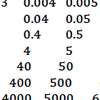### How Can I Support the Development of Early Number Sense and Place Value?

##### Age 3 to 11

This article for primary teachers expands on the key ideas which underpin early number sense and place value, and suggests activities to support learners as they get to grips with these ideas.### Activities on the Gattegno Chart

##### Age 5 to 11

In this article, Alf outlines six activities using the Gattegno chart, which help to develop understanding of place value, multiplication and division.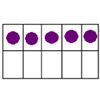### Place Value: the Ten-ness of Ten

##### Age 3 to 11

This article develops the idea of 'ten-ness' as an important element of place value.### The Thousands Game

##### Age 7 to 11 Challenge Level:

Each child in Class 3 took four numbers out of the bag. Who had made the highest even number?### Reach 100

##### Age 7 to 14 Challenge Level:

Choose four different digits from 1-9 and put one in each box so that the resulting four two-digit numbers add to a total of 100.### Repeaters

##### Age 11 to 14 Challenge Level:

Choose any 3 digits and make a 6 digit number by repeating the 3 digits in the same order (e.g. 594594). Explain why whatever digits you choose the number will always be divisible by 7, 11 and 13.### Which Scripts?

##### Age 7 to 11 Challenge Level:

There are six numbers written in five different scripts. Can you sort out which is which?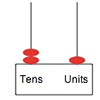### Position

##### Age 5 to 11

One of the key ideas associated with place value is that the position of a digit affects its value. These activities support children in understanding this idea.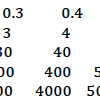### Number Sense and Place Value

##### Age 5 to 11

This feature aims to support you in developing children's early number sense and understanding of place value.### Arrange the Digits

##### Age 11 to 14 Challenge Level:

Can you arrange the digits 1,2,3,4,5,6,7,8,9 into three 3-digit numbers such that their total is close to 1500?### More Upper Primary Activities on Number Sense and Place Value

##### Age 7 to 11

More upper primary number sense and place value tasks.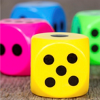### Dicey Operations in Line for Two

##### Age 7 to 11 Challenge Level:

Dicey Operations for an adult and child. Can you get close to 1000 than your partner?